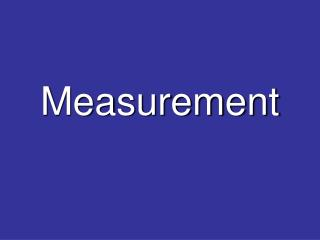DownloadDownload PresentationMeasurement

# Measurement

Download Presentation## Measurement

- - - - - - - - - - - - - - - - - - - - - - - - - - - E N D - - - - - - - - - - - - - - - - - - - - - - - - - - -
##### Presentation Transcript

1. Measurement

2. Area of Square • Square A = l x w A = 4 x 4 W = 4 A = 16 units2 L = 4

3. Area of Rectangle • Rectangle A = l x w A = 6 x 4 W = 4 A = 24 units2 L = 6

4. Area of a Triangle • Triangle A = ½ (b x h) A = ½ (4 x 4) h = 4 A = ½ (16) A = 8 units2 b = 4

5. Finding the Area of Regular Shapes • Square A = l x w • Rectangle A = l x w • Triangle A = ½ (b x h)

6. Finding the Area of Composite Shapes

7. Becomes………. + +

8. Becomes………. + +

9. We can use this strategy to help us find the area of a parallelogram by changing the location of the end triangles + + + =

10. Can we apply the same strategies to finding the area of a trapezoid……? b1 Where b1 and b2 represent the parallel sides h is the height h b2

11. Using graph paper, draw a trapezoid with the following units… Now, see if you can break it apart into different shapes, rearrange and find a new formula…… b1 = 3 h= 4 b2 = 5

12. Copy, Reflect, and Translate the trapezoid……what shape do you have now???? Do you know the formula for this new shape? How can you modify it for a TRAPEZOID? b1 = 3 h= 4 b2 = 5

13. Now we have a parallelogram…. only it’s 2x’s as big as the trapezoid b1 = 3 h= 4 h= 4 b2 = 5 b2 = 5

14. b2 = 5 b1 = 3 h= 4 b2 = 5 b1 = 3 The are of this PARALLELOGRAM is A = base x height A = (b1 + b2) x height

15. b2 = 5 b1 = 3 h= 4 b2 = 5 b1 = 3 It doesn’t matter which type of trapezoid you use, copy, rotate and put the 2 together and you get a parrallelogram!!!! A = ½ [ (b1 + b2) x h ]

16. b2 = 7 b1 = 3 h= 4 b2 = 7 b1 = 3 But, because the TRAPEZOID is only half the size of the parallelogram, we need to take half of the area….. A = (b1 + b2) x h 2

17. b2 = 6 b1 = 3 h= 4 b2 = 6 b1 = 3 But, because the TRAPEZOID is only half the size of the parallelogram, we need to take half of the area….. A = (b1 + b2) x h 2

18. A = ½ [ (b1 + b2) x h ] Or A = [ (b1 + b2) x h ] / 2 Or A = (b1 + b2) x h 2

19. Is there another way???? b1 = 4 cm 3 cm b2 = 7 cm

20. We can divide the trapezoid into 2 Triangles b1 = 4 cm 3 cm 3 cm b2 = 7 cm

21. b1 = 4 cm 3 cm b2 = 7 cm 3 cm You should notice that the height should be the same for both triangles.

22. b1 = 4 cm 3 cm b2 = 7 cm 3 cm Area = Triangle 1 + Triangle 2 A = b1 x h + b2 x h2 2

23. b1 = 6 h= 8 b2 = 10 Find the area of the following Trapezoids. b1 = 2 h= 6 b2 = 8